Updating search results...

# 12 Results

View
Selected filters:
• Learn Zillion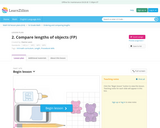Rating
0.0 stars

Lesson objective: Extend prior knowledge about comparing two objects and develop the understanding that comparing lengths of objects requires taking both endpoints into account.
This lesson helps to build understanding that comparing lengths of objects requires taking both endpoints into account. Images are used here because they support the procedural skills of comparing lengths of objects. This work develops students' understanding that both endpoints must be taken into account when comparing lengths of objects.
Students bring prior knowledge of comparing two objects describing measureable attributes of objects, such as length or weight (Grade K, Unit 8, K.MD.1D), and directly compare two objects with a measureable attribute in common, to see which object has "more of"/"less of" the attribute, and describe the difference (Grade K, Unit 8, K.MD.1D).
This prior knowledge is extended to taking both endpoints into account as students must determine which pieces of licorice are longer. A conceptual challenge students may encounter is considering only one pair of endpoints when comparing objects.
The concept is developed through work with linear models such as ribbons, pieces of paper, or string which allow students to physically match up and align objects at the same endpoints and compare the other endpoints.
This work helps students deepen their understanding of numbers because when students compare length they can extend this to number comparisons. It also extends understanding of equivalence and non-equivalence.
Students engage in Mathematical Practice 2 (Students reason quantitatively and abstractly as they use measurements of others to compare their results), Mathematical Practice 3 (Construct viable arguments and critique the reasoning of others as students compare the measurements of others and construct a viable argument as to why the process produced correct or incorrect responses), and Mathematical Practice 6 (Attend to precision). Students will use mathematics vocabulary properly when discussing problems. Carefully showing the steps in solving comparison problems.
Key vocabulary:
lengthlongshortmeasureendpoint

Subject:
Mathematics
Material Type:
Lesson Plan
Simulation
Provider:
Learn Zillion
03/20/2018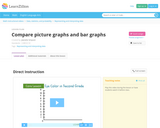Restricted Use
Rating
0.0 stars

In this lesson you will learn the similarities and differences between bar and picture graphs by creating each graph using the same information.

Subject:
Mathematics
Material Type:
Diagram/Illustration
Lesson Plan
Provider:
Learn Zillion
04/05/2016Restricted Use
Rating
0.0 stars

This lesson teaches students to understand figurative language and how it effects meaning.   The students will read a nonfiction piece on The Great Chicago Fire.  Students can then work with a partner or small group to answer the questions about the figurative language.

Subject:
English Language Arts
Material Type:
Formative Assessment
Lesson Plan
Provider:
Learn Zillion
04/20/2016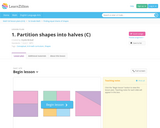Rating
0.0 stars

Lesson objective: Understand that shapes can be partitioned into two equal shares that we call halves, and that those two shares together equal one whole.
Students bring prior knowledge of modeling and composing shapes from Grade K, Unit 15, K.G.B.5. This prior knowledge is extended to introduce part-whole relationships of shapes as students partition shapes to demonstrate equal shares that we call halves. A conceptual challenge students may encounter is partitioning shapes unequally because they think that any two parts equal halves.
The concept is developed through work with basic shapes, like rectangles, which support an introduction into thinking about fractional parts of a whole (something students will need to know in future grades).
This work helps students deepen their understanding of equivalence because when a whole is partitioned into halves, the halves must be equal parts of the whole. Additional evidence of equivalence in these key concepts is that 2 halves equal 1 whole.
Students engage in Mathematical Practice 3 (construct viable arguments and critique the reasoning of others) as they help a student partition her rectangular room into equal shares.
Key vocabulary:
equal shares
Special materials needed:
pattern blocksshape cut-outs

Subject:
Mathematics
Material Type:
Activity/Lab
Lesson Plan
Simulation
Provider:
Learn Zillion
04/10/2017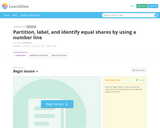Restricted Use
Rating
0.0 stars

Big Ideas: A fraction represents the division of a whole into equal shares. The denominator is represented by the number of segments on a number line between two whole numbers.
This lesson builds on students' work with partitioning area models of wholes into equal shares. This task requires students to recognize whether number lines were partitioned correctly, justify their reasoning, and partition number lines on their own. Students will be building the foundations of fraction sense on a number line by representing, partitioning, and labeling equal shares. The mathematical concepts in this lesson build toward students' future work with composing, decomposing, and identifying specific fractions on number lines as well as future work with comparing and equivalent fractions.
Vocabulary: number line, line segments, fraction, numerator, denominator, halves, thirds, fourths, sixths, eighths

Subject:
Mathematics
Material Type:
Diagram/Illustration
Formative Assessment
Lesson Plan
Provider:
Learn Zillion
06/16/2015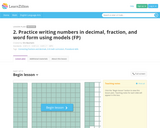Restricted Use
Rating
0.0 stars

Students will practice writing decimals, fractions, and the word form associated with them.
Mathematical Practice 4 - Model with Mathematics - is focused on in this lesson as they represent fractions and decimals in numerous forms and with models.
The students will watch a short video and then the teacher will lead them through discussion and activities.

Subject:
Mathematics
Material Type:
Formative Assessment
Lesson Plan
Provider:
Learn Zillion
04/20/2016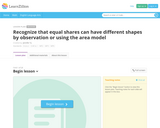Restricted Use
Rating
0.0 stars

Big Ideas: A fraction describes the division of wholes into equal shares. Equal shares have the same area or amount, but do not have to be the same shape.
This lesson builds on students' work with partitioning circles and rectangles into two, three, and four equal shares. This task uses a scenario in which students share a birthday cake. Students will be presented with kids who each want to share their cake differently and asked to justify which kids are sharing equally. Students will be building their foundational knowledge of fractions by representing, labeling, and justifying equal shares. The mathematical concepts in this lesson build toward students' future work with naming fractions, comparing fractions, and equivalent fractions.
Vocabulary: fraction, equal shares, halves, thirds, fourths, sixths, eighths

Subject:
Mathematics
Material Type:
Diagram/Illustration
Formative Assessment
Lesson Plan
Provider:
Learn Zillion
06/16/2015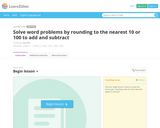Restricted Use
Rating
0.0 stars

Big Ideas: Rounding is an appropriate strategy for solving problems and estimating. Numbers can be decomposed and recomposed to add and subtract.
This lesson builds on student knowledge of place value of whole numbers less than or equal to 1000. This task uses a range of weights for endangered animals for students to apply rounding principles to make totals of 1000 and 2000 using addition and subtraction strategies. This builds conceptual understanding of rounding to build upon for practical use for problem solving using addition, subtraction, multiplication, and division.
Vocabulary: base-ten, expanded form, place value, accurate, complements, commutative property, partial sums

Subject:
Mathematics
Material Type:
Diagram/Illustration
Formative Assessment
Lesson Plan
Provider:
Learn Zillion
06/16/2015Rating
0.0 stars

Lesson objective: Explore the characteristics that define different shapes and the names of those shapes.
Students bring prior knowledge of naming shapes regardless of their orientations or overall size from (K.G.2). This prior knowledge is extended to defining and non-defining attributes as students determine what things are true for all triangles, all squares, etc. A conceptual challenge students may encounter is thinking attributes such as size, color, and orientation are what determine a shape.
The concept is developed through a sorting activity, which encourage students to consider common attributes and predict which shape can be added to a circle based on a defining attribute.
This work helps students deepen their understanding of numbers because shapes can be defined by number of sides, angles, and faces.
Students engage in Mathematical Practice 3 (Construct viable arguments and critique the reasoning of others) as they use clear definitions with peers and define attributes and provide a viable argument reasoning why a shape lacks specific attributes.

Subject:
Mathematics
Material Type:
Diagram/Illustration
Lesson Plan
Reference Material
Simulation
Provider:
Learn Zillion
04/10/2017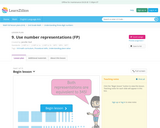Rating
0.0 stars

Lesson objective: Represent numbers in two or more equivalent ways.
This lesson helps to build fluency with place value concepts. Drawing is used here because it supports students moving on from concrete (the base ten blocks) to more representational in the concept. This work develops students' understanding that three-digit numbers can be represented in different, but equivalent ways.
Students engage in Mathematical Practice 7 (Look for and make use of structure) as they understand that three-digit numbers have more than one way to be represented; this comes with repeated experimentation with our place value system.
Key vocabulary:
hundredsplace valuerepresentionstens

Subject:
Mathematics
Material Type:
Lesson Plan
Provider:
Learn Zillion
03/20/2018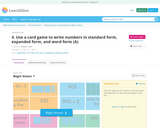Rating
0.0 stars

Lesson objective: Apply knowledge of ways to name numbers.
This lesson provides an opportunity for students to apply their knowledge and understanding of naming numbers to a mathematical situation. Students are asked to help the teacher complete her set of game cards by coming up with equivalent names for numbers.
Key Concept students will use:
Representing a whole number in different ways (including base ten numerals, number-names, and expanded form) does not change its value.
Skills students will use:
Understand that the three digits of a three-digit number represent the amount of hundreds, tens, and ones (Grade 2, Unit 6)Write three-digit numbers in expanded formWrite three-digit numbers in word formWrite three-digit numbers in standard form
Students engage in Mathematical Practice 7 (Look for and make use of structure) as they look for patterns in the way we name numbers to name numbers they have not seen before.

Subject:
Mathematics
Material Type:
Lesson Plan
Provider:
Learn Zillion
03/20/2018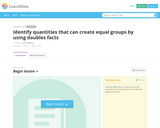Restricted Use
Rating
0.0 stars

Big Ideas: Doubles facts can help determine numbers that can be divided equally. Even numbers create equal shares. This task builds on students' work with addition 'doubles' facts. In this lesson students will hear a story about how Ajani and Ian share everything equally. Students will determine whether they can share a given number of tokens to play arcade games. Students will group using manipulatives and learn what makes a number even or odd by splitting a number into two equal groups. Students will conceptually understand the patterns that even and odd numbers make. The mathematical concepts in this lesson build towards students' future work with building arrays, repeated addition, and multiplication.

Subject:
Mathematics
Material Type:
Diagram/Illustration
Formative Assessment